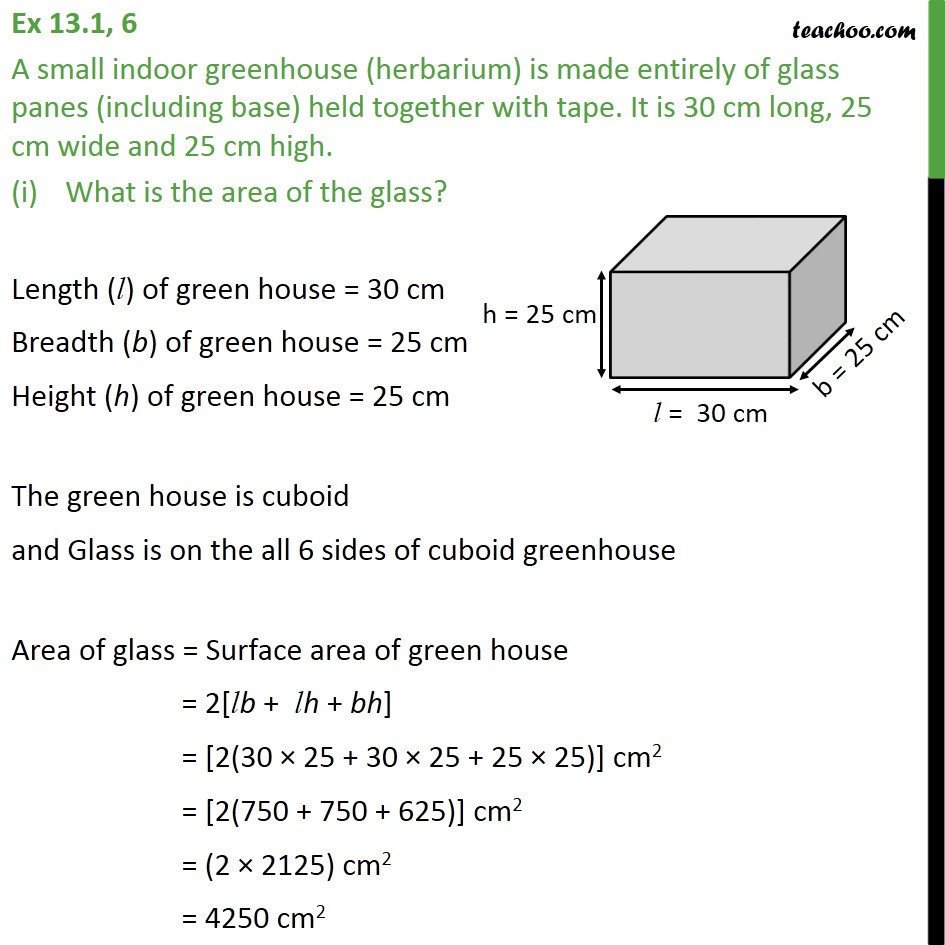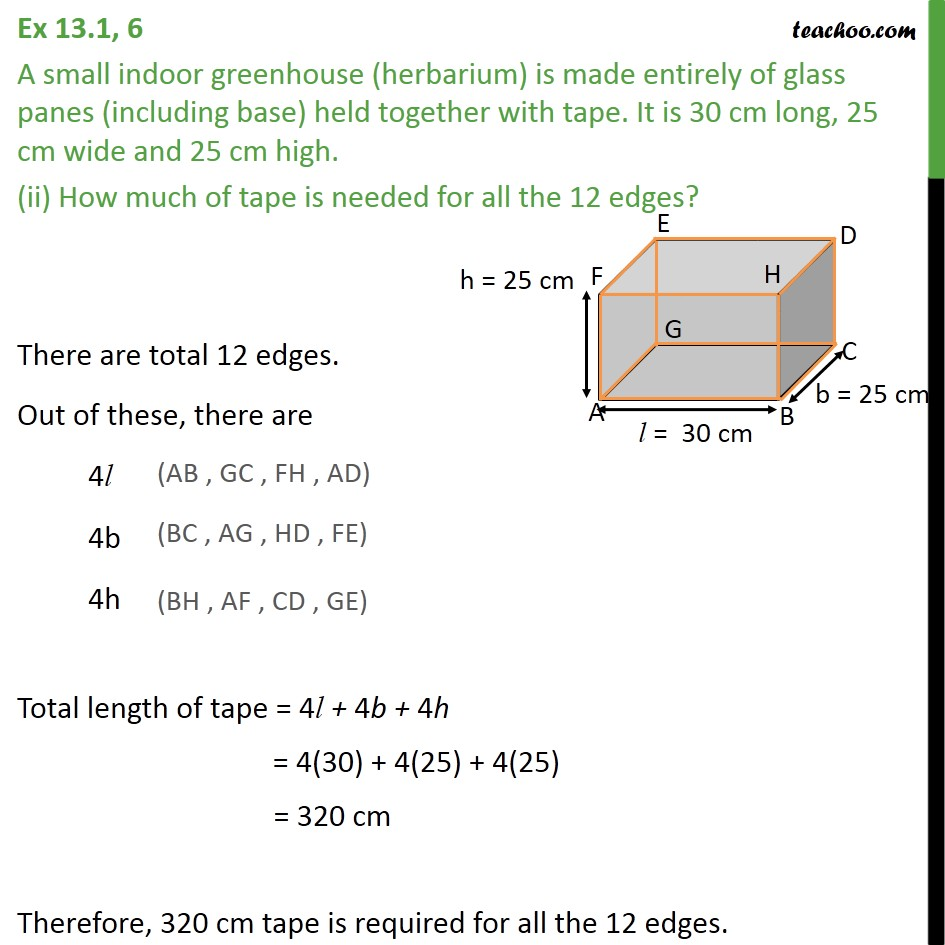Area of Cube and Cuboid

Chapter 11 Class 9 Surface Areas and Volumes
Serial order wiseLearn in your speed, with individual attention - Teachoo Maths 1-on-1 Class

### Transcript

Question 6 A small indoor greenhouse (herbarium) is made entirely of glass panes (including base) held together with tape. It is 30 cm long, 25 cm wide and 25 cm high. What is the area of the glass? Length (l) of green house = 30 cm Breadth (b) of green house = 25 cm Height (h) of green house = 25 cm The green house is cuboid and Glass is on the all 6 sides of cuboid greenhouse Area of glass = Surface area of green house = 2[lb + lh + bh] = [2(30 25 + 30 25 + 25 25)] cm2 = [2(750 + 750 + 625)] cm2 = (2 2125) cm2 = 4250 cm2 Therefore, the area of glass is 4250 cm2. Question 6 A small indoor greenhouse (herbarium) is made entirely of glass panes (including base) held together with tape. It is 30 cm long, 25 cm wide and 25 cm high. (ii) How much of tape is needed for all the 12 edges? There are total 12 edges. Out of these, there are 4l 4b 4h Total length of tape = 4l + 4b + 4h = 4(30) + 4(25) + 4(25) = 320 cm Therefore, 320 cm tape is required for all the 12 edges.